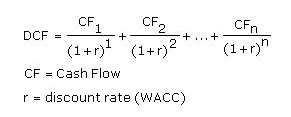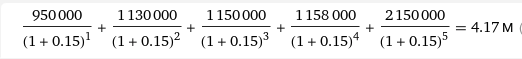# What is the Discounted Cash Flow Startup Valuation Method?

The meta image of this post is the result of using the prompt "A photograph of a seagull flying over a forest of avocados vaporware 4K" in DALL-E

# What is it?

The Discounted Cash Flow valuation method is a way to determine the intrinsic value of a startup based on its expected future cash flows.

The idea behind the DCF method is that the value of an asset or business is the sum of all of its future cash flows, discounted back to their present value (so future earnings are worth less today than in the future). It can be applied for both established companies and startups, if not with some caution and tweaking for the latter.

This approach might be useful for a startup because it is not uncommon for this kind of business to not have realized any profit yet and thus lack historical performance.

# How it works

This method is based on a very simple formula:What each part of the equation means will become clear in no time:

## Free Cash Flow (CF/FCF)

The Free Cash Flows (CF or FCF) can be seen as the future financial achievements of the firm, which are used to determine the value of the startup today. They represent the available cash in each period of time of the formula (normally a year) after all potential liabilities have been fulfilled.

The formula for the FCF is calculated as:

```FCF(i) = EBIT - T - I - D - IWC
```

And again to explain what each piece means:

• EBIT: It stands for Earnings before Interest and Taxes. It represents the startup's expected profit before interest and taxes are deducted.
• T: Taxes, variable each year depending on profit and other factors.
• I: Investments, such as property, plant, and equipment.
• D: Depreciation of the assets.
• IWC: Investments in Working Capital. Those are investments in cash and current assets.

## Discount factor ®

The discount factor is a magnitude that helps to determine today's value of future cash flows. It is calculated using the Weighted Average Cost of Capital or WACC. Calculating this magnitude entails quite a bit of complexity and is outside of the scope of this article.

The WACC is a way to define the risk an investor is taking when putting money into a startup. It is calculated as a percentage. The higher the WACC, the higher the risk and therefore the lower the startup's valuation.

The Discount Factor for a given period of time (like for the FCF) is calculated as:

```Discount factor = 1 / (1 + WACC in %) ^ number of time period
```

The number of time periods is the year which the discount factor is calculated for.

## Terminal Value

The Discounted Cash Flow method is normally calculated with a specific time frame in mind. Say for example for a period of 3 years, the DCF formula looks like this:

```DCF = (FCF(1) / (1 + WACC(1))^1) + (FCF(2) / (1 + WACC(2))^2) + (FCF(3) / (1 + WACC(3))^3)
```

But that formula only accounts for the potential earnings of the startup in the first, second and third year. In order to calculate what are the earnings in the period thereafter, we calculate the Terminal Value.

The Terminal Value formula is:

```Terminal Value = FCF after year 3 * (1 + growth rate) / ((WACC(3) – growth rate)
```
```FCF after year 3 = FCF last proyected period (year 3) * (1 + growth factor)
```

The growth factor can be calculated conservatively using the inflation percentage, or optimistically by using the projected yearly growth rate of the startup.

# Example

Whew! quite a snack of information to digest beforehand. To calculate the DCF method of any business we need to do a bit of due diligence and create financial projections of the firm, regardless of whether it's a startup or an established business.

Let's say we want to grasp the valuation of a promising startup in the PropTech industry called Rompetechos ("ceiling-breaker" in Spanish, a nod to a famous Spanish comedy comic strip about a man who has very bad eyesight). We want to use the DCF valuation method to arrive at a sensible valuation number.

We hire a financial consulting firm that will perform an exhaustive analysis of the company and provide us with the different projections we need for the next 5 years. We receive the following table:

2022 2023 2024 2025 2026 Terminal Value
EBIT 1.3m 1.6m 1.8m 2.3m 3m
Tax (25%) 325K 400K 450K 575K 750K
Net Profit after Taxes 975K 1.2M 1.35M 1.73M 2.25M
Investments -100K -50K -60K -200K -120K
Depreciation
Investment in Working Capital -25K -70K -200K -150K -100K
Free Cash Flow 950K 1.13M 1.15M 1.58M 2.15M 16.87M
Growth Rate (2%)
WACC (15%)
Discount Period 1 2 3 4 5 5
Discount Factor 0.87 0.76 0.66 0.57 0.5 0.5

We are not taking into account the Terminal Value for this case because as a startup, adding the potential cashflows after the fifth year seems too far to be representative.

So applying the DCF formula we arrive at a valuation of:Have fun!